# Difference between revisions of "0708-1300/the unit sphere in a Hilbert space is contractible"

Let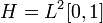$H=L^2[0,1]$ and define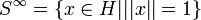$S^{\infty}=\{x\in H| ||x||=1\}$

Claim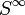$S^{\infty}$ is contractible

Proof

For any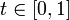$t\in[0,1]$ and any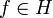$f\in H$ define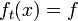$f_t(x)= f$ for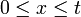$0\leq x \leq t$ and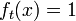$f_t(x)=1$ for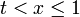$t. Observe that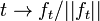$t\rightarrow f_t/||f_t||$ is continuous and gives the desired retraction to the point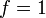$f=1$.

This proof only works in separable Hilbert spaces? Is the unit ball in a non-separable Hilbert space contractible?

The answer seems to be YES see Spheres in infinite-dimensional normed spaces are Lipschitz contractible